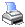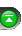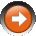﻿ C# Method (Functions) Reference
 NinjaScript > Educational Resources > Tips > C# Method (Functions) ReferenceThe Microsoft .NET environment has a rich class library that you can access when developing custom indicators and strategies. There is a plethora of information available online and in print that details class libraries in great depth. Below are quick links to the Microsoft Developers Network for some of the basic classes whose functionality you may harness when developing in NinjaScript.

Complete list of classes in the Microsoft .NET environment.

MSDN (Microsoft Developers Network) C# Language Reference

Keywords

Operators

Arrays

System.Math

Provides constants and static methods for trigonometric, logarithmic, and other common mathematical functions.

Full list of member of the System.Math class.

 // Example of the Max method of the System.Math class int myInteger = Math.Max(10, 20); Print("The larger value between 10 and 20 is " + myInteger.ToString());

System.DateTime

Represents an instant in time, typically expressed as a data and time of day.

Full list of members of the Sytem.DateTime structure.

 // Example of the Now property member of the System.DateTime structure DateTime startTime = DateTime.Now; Print("Time elapsed is " + DateTime.Now.Subtract(startTime).TotalMilliseconds.ToString() + " milliseconds.");

System.String

Represents text; that is, a series of unicode characters.

Full list of members of the System.String class.

 // Example of the ToUpper() method of the System.String class string myString = "ninjatrader"; Print("The following word is in uppercase " + myString.ToUpper());